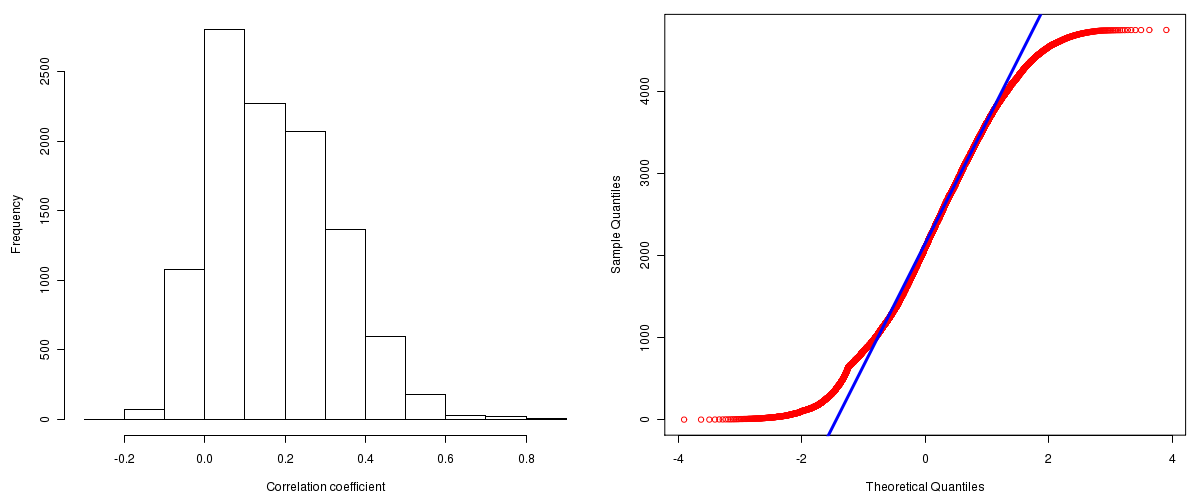Glioblastoma Multiforme: Correlations between copy number and mRNA expression
Maintained by John Zhang (Dana-Farber Cancer Institute)
Overview
Introduction

A TCGA sample is profiled to detect the copy number variations and expressions of genes. This pipeline attempts to correlate copy number and expression data of genes across samples to determine if the copy number variations also result in differential expressions. This report contains the calculated correlation coefficients based on measurements of genomic copy number (log2) values and intensity of the expressions of the corresponding feature across patients. High positive/low negative correlation coefficients indicate that genomic alterations result in differences in the expressions of mRNA the genomic regions transcribe.

Summary

The correlation coefficients in 10, 20, 30, 40, 50, 60, 70, 80, 90 percentiles are -0.0046, 0.0335, 0.0702, 0.11046, 0.1566, 0.2039, 0.251, 0.30614, 0.38091, respectively.

Results
Correlation results

Number of genes and samples used for the calculation are shown in Table 1. Figure 1 shows the distribution of calculated correlation coefficients and quantile-quantile plot of the calculated correlation coefficients against a normal distribution. Table 2 shows the top 20 features ordered by the value of correlation coefficients.

Table 1.  Counts of mRNA and number of samples in copy number and expression data sets and common to both

Category Copy number Expression Common
Sample 537 529 470
Genes 29390 12043 10944

Figure 1.  Summary figures. Left: histogram showing the distribution of the calculated correlations across samples for all Genes. Right: QQ plot of the calculated correlations across samples. The QQ plot is used to plot the quantiles of the calculated correlation coefficients against that derived from a normal distribution. Points deviating from the blue line indicate deviation from normality.Table 2.  Get Full Table Top 20 features (defined by the feature column) ranked by correlation coefficients

feature r p-value q-value chrom start end geneid
TSFM 0.8744 0 0 12 56462826 56476784 10102
SEC61G 0.8656 0 0 7 54787434 54794433 23480
METTL1 0.8609 0 0 12 56448521 56452522 4234
CDK4 0.8419 0 0 12 56428270 56432431 1019
TSPAN31 0.8355 0 0 12 56425051 56428293 6302
LANCL2 0.8354 0 0 7 55400635 55468929 55915
MRPS17 0.8296 0 0 7 55987105 55990528 51373
SLC35E3 0.8213 0 0 12 67426203 67446120 55508
DCTN2 0.8068 0 0 12 56210361 56227245 10540
MDM2 0.7911 0 0 12 67488247 67520481 4193
ECOP 0.7797 0 0 7 55505801 55607641 81552
CTDSP2 0.779 0 0 12 56499977 56526789 10106
CYP27B1 0.7702 0 0 12 56442384 56447243 1594
CCT6A 0.7698 0 0 7 56086872 56099176 908
OS9 0.7696 0 0 12 56374153 56401607 10956
ETNK2 0.7687 0 0 1 202366814 202387645 55224
KLHL9 0.7554 0 0 9 21321020 21325371 55958
CHIC2 0.7539 0 0 4 54570713 54625545 26511
MDM4 0.7536 0 0 1 202752189 202808458 4194
EGFR 0.7491 0 0 7 55054219 55242525 1956
Methods & Data
Input

Gene level (TCGA Level III) expression data and copy number data of the corresponding loci derived by using the CNTools package of Bioconductor were used for the calculations. Pearson correlation coefficients were calculated for each pair of genes shared by the two data sets across all the samples that were common.

Correlation across sample

Pairwise correlations between the log2 copy numbers and expressions of each gene across samples were calculated using Pearson correlation.